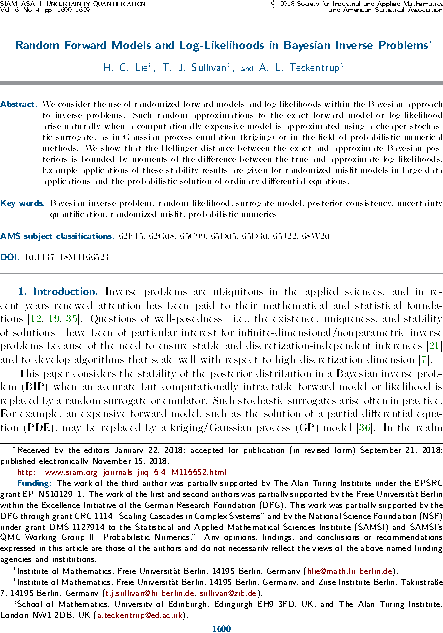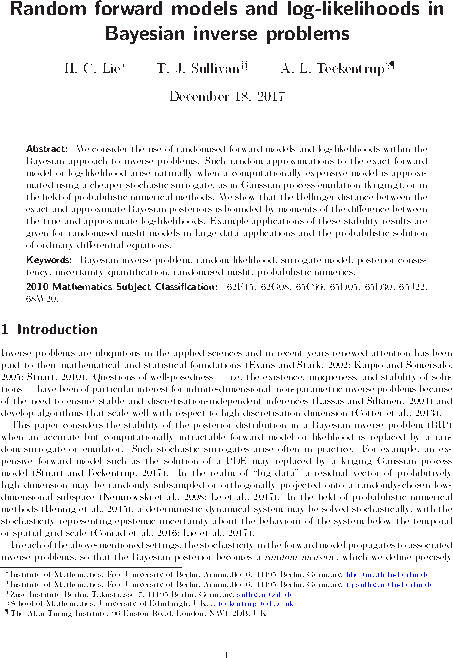# Tim Sullivan

### #teckentrup

Clear Search### Random Bayesian inverse problems in JUQ

The article “Random forward models and log-likelihoods in Bayesian inverse problems” by Han Cheng Lie, Aretha Teckentrup, and myself has now appeared in its final form in the SIAM/ASA Journal on Uncertainty Quantification, volume 6, issue 4. This paper considers the effect of approximating the likelihood in a Bayesian inverse problem by a random surrogate, as frequently happens in applications, with the aim of showing that the perturbed posterior distribution is close to the exact one in a suitable sense. This article considers general randomisation models, and thereby expands upon the previous investigations of Stuart and Teckentrup (2018) in the Gaussian setting.

H. C. Lie, T. J. Sullivan, and A. L. Teckentrup. “Random forward models and log-likelihoods in Bayesian inverse problems.” SIAM/ASA Journal on Uncertainty Quantification 6(4):1600–1629, 2018. doi:10.1137/18M1166523

Abstract. We consider the use of randomised forward models and log-likelihoods within the Bayesian approach to inverse problems. Such random approximations to the exact forward model or log-likelihood arise naturally when a computationally expensive model is approximated using a cheaper stochastic surrogate, as in Gaussian process emulation (kriging), or in the field of probabilistic numerical methods. We show that the Hellinger distance between the exact and approximate Bayesian posteriors is bounded by moments of the difference between the true and approximate log-likelihoods. Example applications of these stability results are given for randomised misfit models in large data applications and the probabilistic solution of ordinary differential equations.

Published on Monday 10 December 2018 at 12:00 UTC #publication #bayesian #inverse-problems #juq #prob-num #lie #teckentrup### Random Bayesian inverse problems

Han Cheng Lie, Aretha Teckentrup, and I have just uploaded a preprint of our latest article, “Random forward models and log-likelihoods in Bayesian inverse problems”, to the arXiv. This paper considers the effect of approximating the likelihood in a Bayesian inverse problem by a random surrogate, as frequently happens in applications, with the aim of showing that the perturbed posterior distribution is close to the exact one in a suitable sense. This article considers general randomisation models, and thereby expands upon the previous investigations of Stuart and Teckentrup (2018) in the Gaussian setting.

Abstract. We consider the use of randomised forward models and log-likelihoods within the Bayesian approach to inverse problems. Such random approximations to the exact forward model or log-likelihood arise naturally when a computationally expensive model is approximated using a cheaper stochastic surrogate, as in Gaussian process emulation (kriging), or in the field of probabilistic numerical methods. We show that the Hellinger distance between the exact and approximate Bayesian posteriors is bounded by moments of the difference between the true and approximate log-likelihoods. Example applications of these stability results are given for randomised misfit models in large data applications and the probabilistic solution of ordinary differential equations.

Published on Tuesday 19 December 2017 at 08:30 UTC #preprint #bayesian #inverse-problems #lie #teckentrup